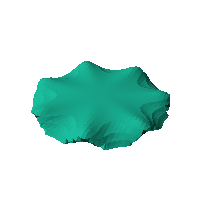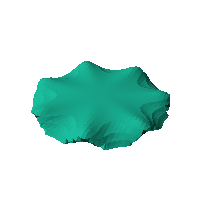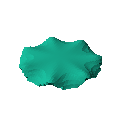Blob Farm# blob10The blob in all its glory:Experiments with making a rippled disk.Click on the snapshot to download the blob's stl file.Octave Code:
```1; # Prevent Octave from thinking that this is a function
# though one is defined here

function w = f(x2,y2,z2,c,r,e)
x  = (x2-c(1))/r(1);
y  = (y2-c(2))/r(2);
z  = (z2-c(3))/r(3);
zp = z - 0.002*sin(7*atan2(x,y)).*(x.^2+y.^2).^2.*z;
# function at origin must be <0, and >0 far enough away.  w=0 defines the surface
w = ((2*zp-2).^4+(0.2*x.*((zp*0.5).^2+1)).^2+(0.2*y.*((zp*0.5).^2+1)).^2);
#w = x.^2+y.^2+z.^2-1.0;
w = w- 0.75;
endfunction;

```
GNU Octave